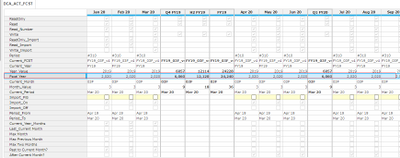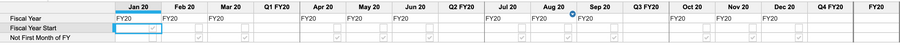# How to obtain start month of a financial year?Hello Experts,

I'd like to obtain start month of a fiscal year.

What I have now is current fiscal year in number format. And what I want to do is to compare item(time) and start month of a fiscal year, and get boolean for this, if item(time) is larger than start month of a fiscal year.Regards,

Inoue

Tagged:

•It is easier to do calc on a period/ date formatted line item when it comes to calc with time periods.

Can you tweak the formulas to this:

Fiscal Year: PERIOD(CURRENTPERIODSTART())

Not First Month of FY: START() > START(Fiscal Year)

•If I understood your question correctly, you want a flag against months whether that month is first month of a fiscal year or not. If that's the case, try the solution below:

1. Create a line item "Fiscal Year" formatted as time period - Year

2. Use the formula "PERIOD(START())" in the line item

3. Then use "START() = START(Fiscal Year)" to identify whether it's the first month or not```Fiscal Year = PERIOD(START())

Fiscal Year Start = START() = START(Fiscal Year)

Not First Month of FY = START() <> START(Fiscal Year)```
•Hello Anik,

In my purpose, there is already "2020" (number format) specified.

Then month after Apr 20 should have true in boolean, on the other hand, in this case, all month in 2018 and 2019 should become false in boolean.

Could you please suggest another solution for this?

•You don't need to convert the time periods in order to compare. You can compare the time periods directly by saying ITEM(TIME) >PERIOD(CURRENTPERIODEND()) assuming you have current period set up on TIME

•Hi Anik,

**** CURRENTPERIODSTART is so useful! Thanks so much!

Regards,

Inoue